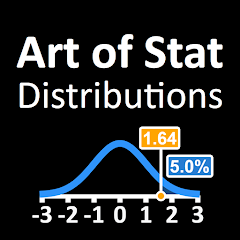# Art of Stat: Distributions

In-app purchases
500+Everyone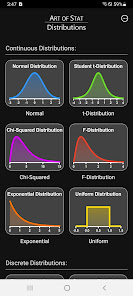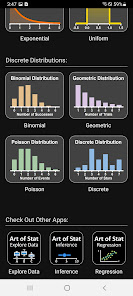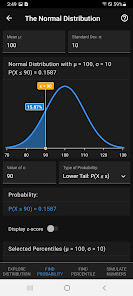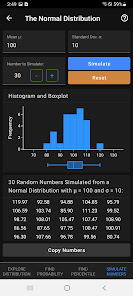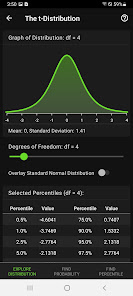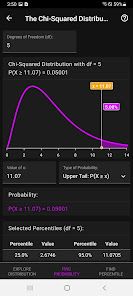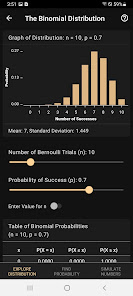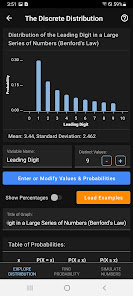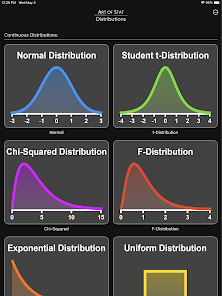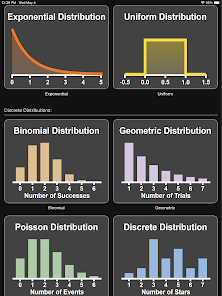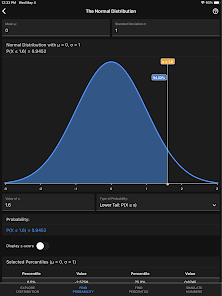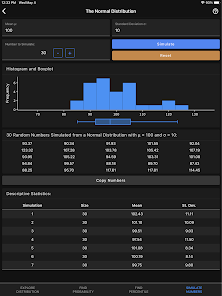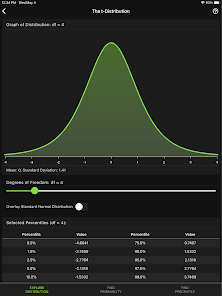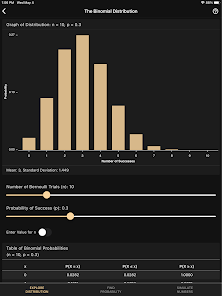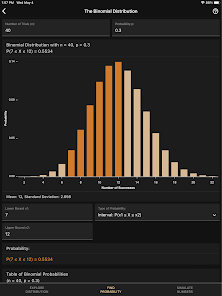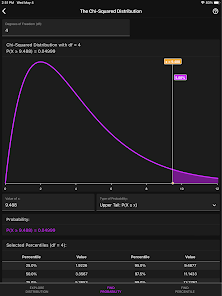Interactively explore and visualize probability distributions via sliders and buttons. Find probabilities or percentiles (two-tailed, upper tail or lower tail) for computing P-values. Find critical values for confidence intervals. Compute the expected value and standard deviation of discrete distributions. Simulate random numbers.

Developed for both students and teachers as a handy tool to visualize and explore probability distributions and their properties. Don't rely on boring and complicated graphing calculators that don't deserve their name. Instead, use the interactive graphics that this app provides to learn about probability distributions and visualize results side-by-side with the numerical solutions.

The app works in offline (airplane) mode and indicates this by changing the background color.

Continuous Distributions implemented so far:
- Normal
- Student's t
- Chi-Squared
- F
- Exponential
- Uniform

Discrete Distributions:
- Binomial
- Geometric
- Poisson